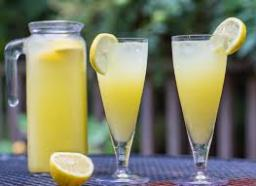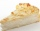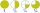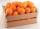How many 1/4 cup servings are in 2 and 1/3 cups of lemonade?

Correct result:

n =  9

#### Solution:We would be pleased if you find an error in the word problem, spelling mistakes, or inaccuracies and send it to us. Thank you!Tips to related online calculators
Need help calculate sum, simplify or multiply fractions? Try our fraction calculator.
Do you want to round the number?

## Next similar math problems:

• Pizza 5You have 2/4 of a pizza and you want to share it equally between 2 people how much pizza does each person get?
• Cake 71/3 of a cake shared with 4 people. What share of the whole cake has each people?
• A bakerA baker has 5 1/4 pies in her shop. She cut the pies in pieces that are each 1/8 of a whole pie. How many pieces of pie does she have?
• ChocolateChildren break chocolate first to third and then every part of another half. What kind got each child? Draw a picture. What part would have received if each piece have halved?
• Product of two fractionsProduct of two fractions is 9 3/5 . If one of the fraction is 9 3/7. Find the other fraction.
• Two dividedTwo divided by nine tenths.
• Fractions 4How many 2/3s are in 6?
• Crates 2One crate will hold 50 oranges. If Bob needs to ship 932 oranges, how many crates will he need?
• A quotientWhat is the quotient of 3/10 divided by 2/4 as a fraction
• Lengths of the poolMiguel swam 6 lengths of the pool. Mat swam 3 times as far as Miguel. Lionel swam 1/3 as far as Miguel. How many lengths did mat swim?
• Fraction to decimalWrite the fraction 3/22 as a decimal.
• In fractionsAn ant climbs 2/5 of the pole on the first hour and climbs 1/4 of the pole on the next hour. What part of the pole does the ant climb in two hours?
• Round 9Round number 0.2375 TO 2 SIGNIFICANT FIGURES
• ZdeněkZdeněk picked up 15 l of water from a 100-liter full-water barrel. Write a fraction of what part of Zdeněk's water he picked.
• Evaluate expressionCalculate the value of the expression z/3 - 2 z/9 + 1/6, for z = 2
• Fraction and a decimalWrite as a fraction and a decimal. One and two plus three and five hundredths
• Mixed2improperWrite the mixed number as an improper fraction. 166 2/3